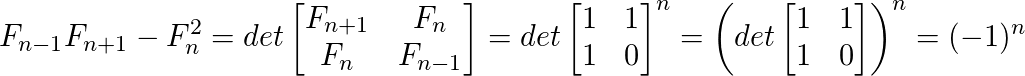Cassini’s Identity

• Difficulty Level : Medium
• Last Updated : 24 Mar, 2021

Given a number N, the task is to evaluate below expression. Expected time complexity is O(1).

f(n-1)*f(n+1) - f(n)*f(n)

Where f(n) is the n-th Fibonacci number with n >= 1. First few Fibonacci numbers are 0, 1, 1, 2, 3, 5, 8, 13, ………..i.e. (considering 0 as 0th Fibonacci number)

Examples :

Input : n = 5
Output : -1
f(5-1=4) = 3
f(5+1=6) = 8
f(5)*f(5)= 5*5 = 25
f(4)*f(6)- f(5)*f(5)= 24-25= -1

Recommended: Please solve it on “PRACTICE ” first, before moving on to the solution.

Although the task is simple i.e. find n-1th, nth and (n+1)-th Fibonacci numbers. Evaluate the expression and display the result. But this can be done in O(1) time using Cassini’s Identity which states that:

f(n-1)*f(n+1) - f(n*n) = (-1)^n

So, we don’t need to calculate any Fibonacci term,the only thing is to check whether n is even or odd.

How does above formula work?
The formula is based on matrix representation of Fibonacci numbers.C/C++

 // C++ implementation to demonstrate working// of Cassini’s Identity #includeusing namespace std;  // Returns (-1)^nint cassini(int n){   return (n & 1) ? -1 : 1;}   // Driver programint main(){     int n = 5;   cout << cassini(n);   return 0;}

Java

 // Java implementation to demonstrate working// of Cassini’s Identity   class Gfg{    // Returns (-1)^n    static int cassini(int n)    {       return (n & 1) != 0 ? -1 : 1;    }       // Driver method    public static void main(String args[])    {         int n = 5;         System.out.println(cassini(n));    }}

Python3

 # Python implementation# to demonstrate working# of Cassini’s Identity   # Returns (-1)^ndef cassini(n):     return -1 if (n & 1) else 1   # Driver program   n = 5print(cassini(n))     # This code is contributed# by Anant Agarwal.

C#

 // C# implementation to demonstrate // working of Cassini’s Identityusing System;  class GFG {      // Returns (-1) ^ n    static int cassini(int n)    {       return (n & 1) != 0 ? -1 : 1;    }        // Driver Code    public static void Main()    {         int n = 5;         Console.Write(cassini(n));    }}  // This code is contributed by Nitin Mittal.



JavaScript



Output :
-1

This article is contributed by Sahil Chhabra. If you like GeeksforGeeks and would like to contribute, you can also write an article using contribute.geeksforgeeks.org or mail your article to contribute@geeksforgeeks.org. See your article appearing on the GeeksforGeeks main page and help other Geeks.